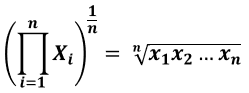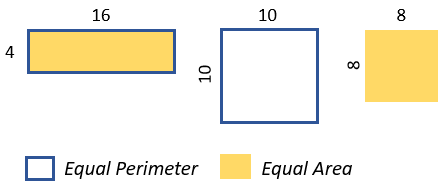# Geometric Mean Calculator

Use this online calculator to easily calculate the Geometric mean for a set of numbers or percentages. Works properly with negative numbers so it can be used to find the geometric mean of returns of investments.

Share calculator:

Embed this tool:
get code

## What is a geometric mean?

The geometric mean, often referred to as the geometric average, is a so-called specialized average and is defined as the n-th root of the product of n numbers of the same sign. If in an arithmetic mean we combine the numbers using the summation operation and then divide by their number, in a geometric mean we calculate the product of the numbers and then take its n-th root. Any time you have several factors contributing to a product, and you want to calculate the "average" of the factors, the answer is the geometric mean.

It is useful in a number of situations where a growth rate is of interest, for example in calculating compound interest rates, financial returns or risk and loses, area and volume averages, in computing indexes such as the U.S. Consumer Price Index (index of inflation), and others. If you are dealing with such tasks, a geometric mean calculator like ours should be most helpful.

## Geometric mean formula

The formula for calculating the geometric mean is:where n is the number of numbers in the set and X1...Xn are the numbers from the first to the n-th. An alternative way to write the formula is (X1 x X2 ... x Xn)^1/n . This formula is used in our calculator.

A geometric approach to explain the formula is through rectangles and squares. If we have a rectangle with sides 4 and 16, the perimeter of the rectangle is the sum of all four sides: 4 + 4 + 16 + 16 = 40. The arithmetic mean of 4 and 16 is 10, and a square with a side of 10 will have the same perimeter as one with sides 4 and 16.

Now, if we take the area of our 4 x 16 rectangle instead, it is the product of 4 and 16 and equals 64. The geometric mean answers the question: what side should a square have so that its area is 64? The answer is 8, which is exactly the geometric mean of 4 and 16.In the image above the perimeter calculation corresponds to finding the arithmetic mean and the area calculation - to finding the geometric mean.

## How to calculate Geometric mean?

Assuming you don't want to use a calculator, obviously. Let's say we have a set of numbers: 1 5 10 13 30 and we want to calculate their arithmetic mean. We would just sum the numbers (1 + 5 + 10 + 13 + 30) and then divide by 5, giving us an arithmetic mean of 11.80. To calculate the geometric mean, we take their product instead: 1 x 5 x 10 x 13 x 30 = 19,500 and then calculate the 5-th root of 19,500 = 7.21. In this case finding the geometric mean is equivalent to raising 19,500 to the 1/5-th power.

Another way to calculate the geometric mean is with logarithms, as it is also the average of logarithmic values converted back to base 10. Let's say you want to calculate the geomean of 2 and 8. It is handy to use log with base 2 here, so 2 = 21 and 8 = 23. The arithmetic mean of the exponents (1 and 3) is 2, so the geometric mean is 22 = 4. This can be verified by our geometric average calculator as well.

As you can see, the geometric mean is significantly more robust to outliers / extreme values. For example, replacing 30 with 100 would yield an arithmetic mean of 25.80, but a geometric mean of just 9.17, which is very desirable in certain situations. However, before settling on using the geometric mean, you should consider if it is the right statistic to use to answer your particular question.

## Geometric mean for negative numbers

From the definition we can see that we can only calculate the geometric mean of positive numbers, or, more precisely, the numbers need to be of the same sign, in order to avoid taking the root of a negative product, which would result in imaginary numbers. However, this doesn't mean we can't work with negative numbers as well. Let's say we have the following relative changes in 3 consecutive years: 8% growth, 10% decline, 11% growth. The total growth at the end is 7.89%, but how do we calculate the average yearly growth rate? 10% would usually be -10%, having a different sign and forbidding us from doing the calculation, but we can do a little trick and express the numbers as proportions, thus 8% growth becomes 1 + 8% x 1 = 1.08, 10% decline becomes 1 - 10% x 1 = 0.9 and 11% growth becomes 1 + 11% x 1 = 1.11. The geometric mean is 1.0256 which equals 2.56% average growth per year.

Our geometric mean calculator handles this automatically, so there is no need to do the above transformations manually. You can also enter the numbers with %, like "2% 10% -10% 8%" and will deal with that as well (it simply strips the %).

## Geometric means with zeros in the dataset

The geometric mean won’t be meaningful if zeros are present in the data. You may be tempted to adjust them in some way so that the calculation can be done. There are same cases when adjustments are justified and the first one is similar to the negative numbers case above. If the data is percentage increases, you can transform them into normal percentage values in the way described for negative numbers. Zeros then become 100% or 1 and the calculation proceeds as normal.

In other cases, zeros mean non-responses and in some cases they can just be deleted before calculation. Of course, this would change the meaning of the reported statistic from applying to the whole dataset to just those people who responded, or those sensors that continue working. Due to these complications, our software would not automatically adjust zeros in any way. You might need to look for another calculator if such an adjustment is desirable.

## Example usage in Finance

When you evaluate an offer for a deposit with compound interest, or the expected returns from an investment strategy, you need to use the geometric average, not the arithmetic average. Let's see a quick example: if you hold money in a mutual fund for two years and it increased the value of its shares by 10% on the first year, and lost 10% on the second year, by using the arithmetic average of (15% - 15%)/2 = 0% you would expect to be where you started, but in fact you would have lost 2.25% of your initial investment (1.15 x 0.85)^1/2 = 0.9775 or 97.75%, losing an average of 1.13% per year.

For a more complex example, let's say you are evaluating a strategy that projects the following return on investment for the next 5 years: 6%, 7%, 8%, -35%, 10%. The arithmetic average would be 0.4% return, but the actual average yearly return over those 5 years would be -2.62%, thus it will lose you money, despite having a positive return in 4 out of 5 years.

Period Starting capital % growth End capital
1-st year \$1,000 6% \$1,060
2-nd year \$1,060 7% \$1,134.2
3-rd year \$1,134.2 8% \$1,224.94
4-th year \$1,224.94 -35% \$796.21
5-th year \$796.21 10% \$875.83

Using the arithmetic average of 0.4% growth per year we expect to see an end capital of \$1020.16, with the geometric average of -2.62% we see exactly \$875.83.

Due to its qualities in correctly reflecting investment growth rates the geometric mean is used in the calculation of key financial indicators such as CAGR.

## Example usage in Social Sciences

Human population growth rate is expressed as a percentage of the current populations, and thus when it needs to be averaged, finding the geometric mean is the proper calculation to do so you can say "the average rate of growth of the population of North America over the past X years was Y%".

In surveys and studies too, the geometric mean becomes relevant. For example, if a survey found that over the years, the economic status of a poor neighborhood is getting better, they need to quote the geometric mean of the development, averaged over the years in which the survey was conducted. The arithmetic mean will not make sense in this case either.

## Other applications

The geometric mean can be useful in many other situations. For example, the geometric mean is the only correct mean when averaging normalized results, which are any results that are presented as ratios to a reference value or values. This is the case when presenting performance with respect to a reference baseline performance, or when computing a single average index from several heterogeneous sources, for example an index composed of indices for health adjusted life expectancy, education years, and infant mortality. In such scenarios the arithmetic or harmonic mean would change the ranking of the results depending on what is used as a reference, while the geomean would preserve them as it is indifferent to the scales used.

The geometric mean is heavily used in geometry. In a right-angled triangle, its altitude is the length of a line extending perpendicularly from the hypotenuse to its 90° vertex. Imagining that this line splits the hypotenuse into two segments, the geometric mean of these segment lengths is the length of the altitude. In another example: the distance to the horizon of a sphere is the geometric mean of the distance to the closest point of the sphere and the distance to the farthest point of the sphere.

It also played a role in deciding on the 16:9 aspect ratio in modern monitors and TV screens. The geometric mean has been used in choosing a compromise aspect ratio between the 4:3 and 2.35:1 ratios as it provided a compromise between them, distorting or cropping both in some sense equally. As you can check using our geomean calculator, the geomean of 1.333(3) and 2.35 is 1.77, which is exactly the ratio between 16 and 9 used in modern 16:9 TV screens and computer monitors.